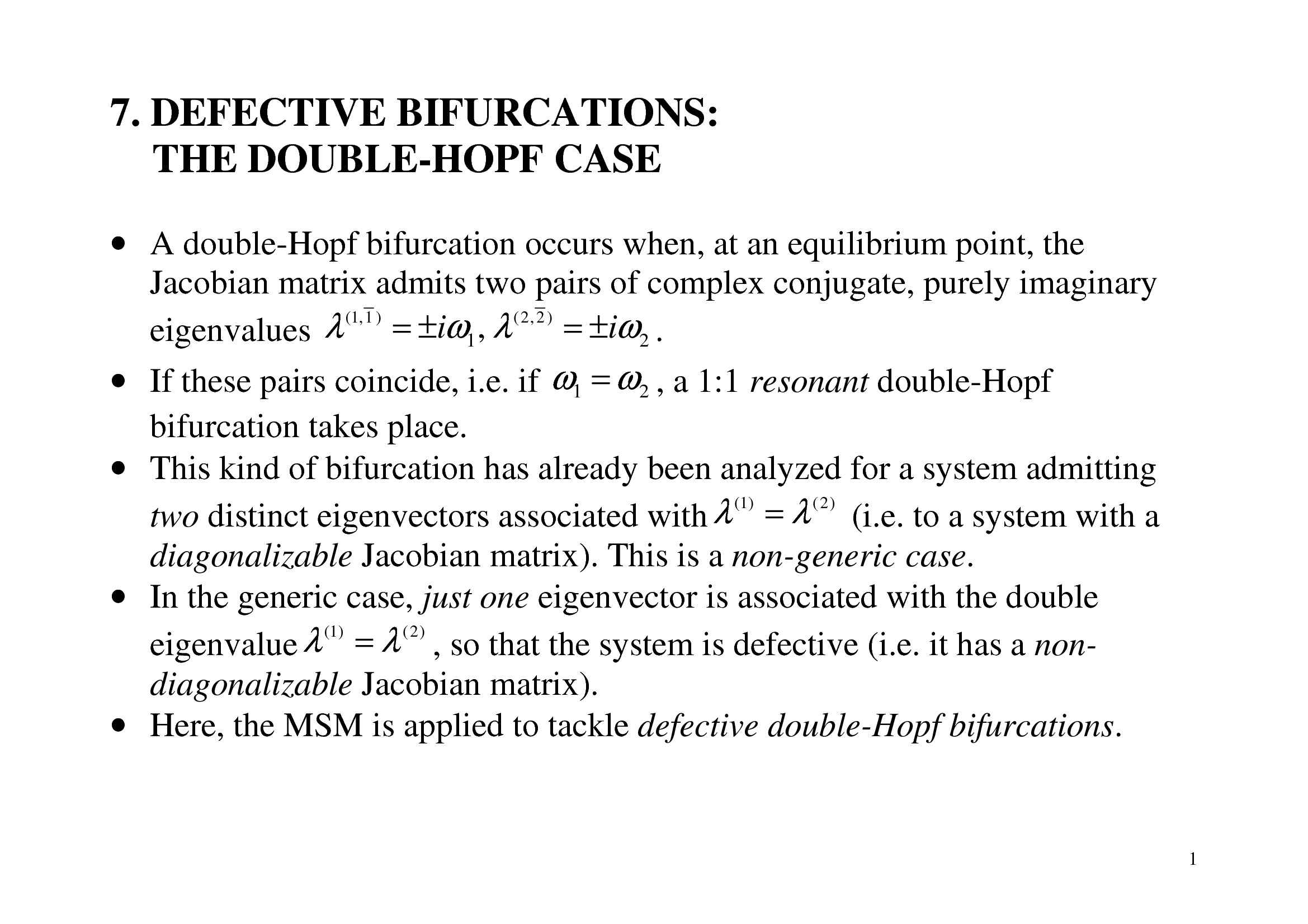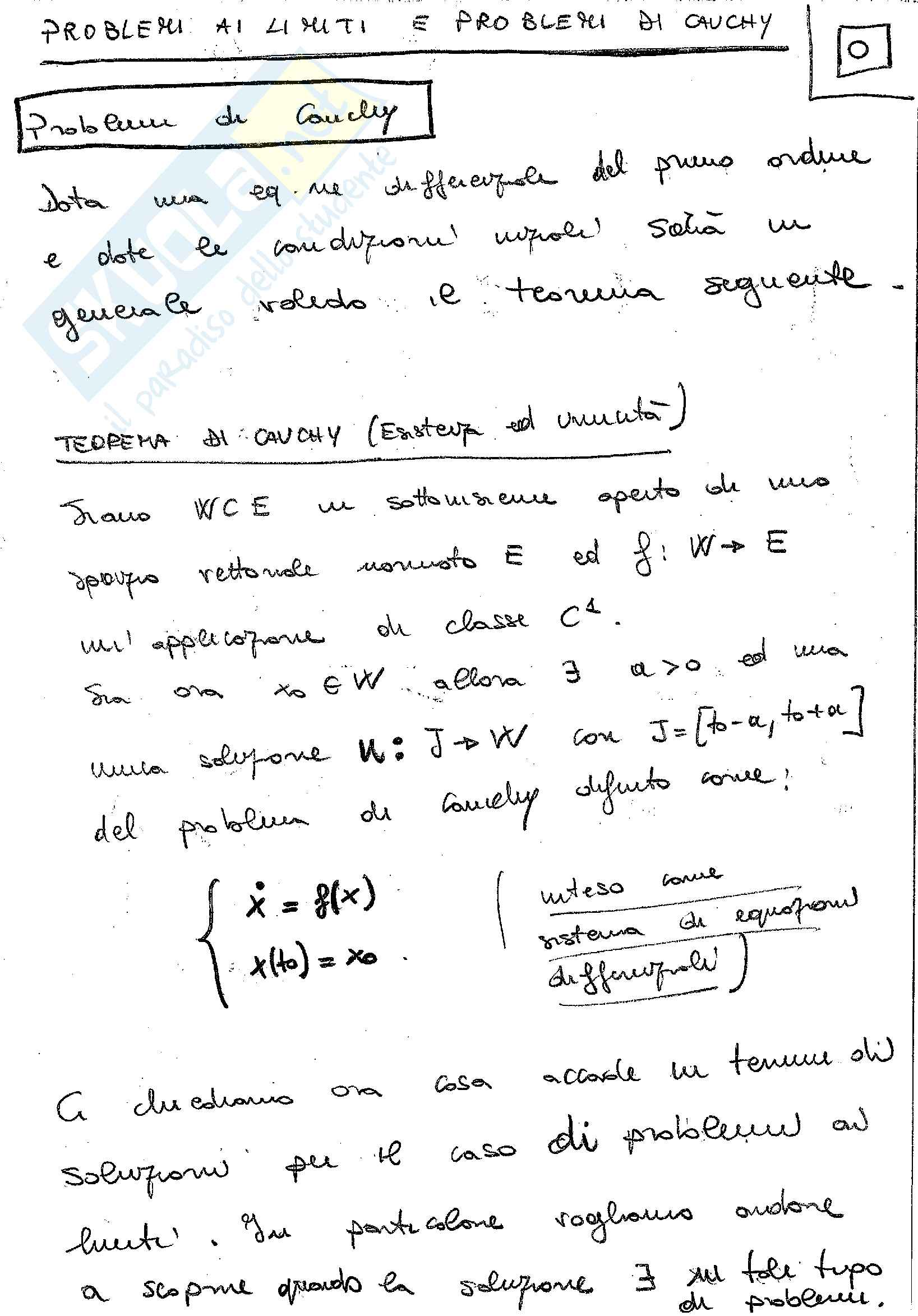Che materia stai cercando?

# Stability and bifurcation of dynamical systems

Stability and bifurcation of dynamical systems
Scope:
- to remind basic notions of Dynamical Systems and Stability Theory;
- to introduce fundaments of Bifurcation Theory, and establish a link with Stability Theory;
- to give an outline of the Center Manifold Method and Normal Form Theory.
Outline:
- General... Vedi di più

Esame di Dynamical systems and bifurcation theory docente Prof. A. Luongo

Anteprima

### ESTRATTO DOCUMENTO

Dynamical system:  2

µ

= +

x x

 = −

 y y

Equilibrium: µ

= ± − =

, 0

x y

## E E

Equilibrium path (or bifurcation diagram): 16

Jacobian matrix at equilibrium:

 

µ

± −

2 0

=  

J E −

0 1

 

µ=0

The equilibrium at is not-hyperbolic; here a bifurcation occurs.

Phase-portraits: 17

Bifurcation of equilibrium points:

= = ∀

x x ( ) F x

( ( ), ) 0

µ

Let be an equilibrium path, i.e. and let

µ µ µ

## E E E

0 = =

x x

: ( )

µ µ µ

be the equilibrium at . We want to analyze changes of

0

E E 0 δ

+

µ µ µ

the system dynamics, when the parameters are perturbed to .

0 0 18

x ( )

µ

Linear dynamics around the perturbed equilibrium point :

## E

δ

= +

x x x

( ) ( ) ( )

t t

µ

E 2

δ δ δ

+ = + +

x x F x F x x x

( ) ( ) ( ( ), ) ( ( ), ) ( ) O( ( ) )

t t t

µ µ µ µ µ

x

## E E E

δ δ

= =

x J x J F x

( ) ( ) ( ), ( ) : ( ( ), )

t t

µ µ µ µ

x

E E E x ( )

µ

Series expansion of the Jacobian matrix at the perturbed point :

## E

=

J F x

( ) ( ( ), )

µ µ µ

x 0 0

E E 2

δ δ

+ + +

F x x F x

[ ( ( ), ) ( ) ( ( ), )] O( )

µ µ µ µ µ µ µ

xx xµ

0 0 0 0 0

## E E E

µ

or, in short: 0 0 δ

= +

: µ

## E E E

µ

x ( )

µ

The local flow around is governed by the eigenvalues of ; these

0

## J

are perturbations of the eigenvalues of .

E 19

Hyperbolic and non-hyperbolic equilibria

0 0

x J

If is hyperbolic, the sign of the real part of the eigenvalues of

o E E

δ

does not change under sufficiently small perturbations , so that the

µ

dynamics remain substantially unaltered. We conclude that the local

phase-portrait at a hyperbolic equilibrium point is structurally stable.

0 0

x J

If is non-hyperbolic, one or more eigenvalues of have zero real

o E E

δ

parts. Therefore, arbitrary small perturbations may lead to

µ

eigenvalues of with (small) positive or negative real parts, thus

## J E

strongly changing the dynamics. We conclude that the local phase-

portrait at a non- hyperbolic equilibrium point is structurally

unstable.

By summarizing:

Bifurcation of equilibrium occurs at points. If the

non-hyperbolic

associated linear system is neutrally stable, then generic perturbations

entail loss of stability of the equilibrium. 20

• Example: Hopf bifurcation

Dynamical system:  2

ρ ρ µ ρ

 = −

( )



θ = 1

Equilibria:

 ρ µ

= ∀

0 ( )

 ρ µ

 = ( )

## NT

Jacobian:  µ on T

2

µ ρ 

= − =

3

J E µ

 -2 on NT 21

Phase-portraits: Linearized system

stable focus center unstable focus

Nonlinear system

Stable focus Stable focus unst. focus + limit cycle

µ<0 µ=0 µ>0 22

The dynamics around points is robust. It is qualitatively the

hyperbolic

o same for linear and nonlinear systems, and does not suffer changes of

parameters.

The dynamics around points is not robust. Adding

non-hyperbolic

o nonlinearities, and/or changing the parameters, may lead to strong

changes. 23

## 4. MULTIPLE BIFURCATIONS FROM A KNOWN PATH

Bifurcations from a known path

We consider an autonomous dynamical system depending on parameters:

## N M

= ∈ ∈

x F x x

( ) ( ( ), ), ,

t t µ µ

We assume to know an equilibrium path (named fundamental path)

=

x x ( )

µ ; we want:

E E of the parameters for which a bifurcation takes

(a) to find the values µ c

place (analysis of critical behavior);

(b) to study the dynamics of the nonlinear system for values of close to

µ

(post-critical behavior).

µ c

For task (a), a linearized analysis is required; for task (b), a nonlinear

analysis is necessary. 24

Local form of the equation of motion

It is convenient to introduce local coordinates:

= −

x x x

( , ) : ( ) ( )

t t

µ µ

## E

x

( , )

t

Here denotes, for a given the deviation of the actual state

µ µ,

from the corresponding equilibrium. Often, the dependence on is

µ

x

=

x x

is written as .

understood, and ( )

t

The corresponding of the equations read:

local form

= + =

x F x x F x

( ) ( ( ) ( ), ) : ( ( ), )

t t t

µ µ µ

## E

or, by omitting the tilde:

= = ∀

x F x F 0 0

( ) ( ( ), ), ( , )

t t µ µ µ 25

In the new variables, the fundamental path appears as trivial:

= ∀

x 0 µ

## E

Geometrical meaning of the local coordinates: 26

Static and dynamic bifurcations

=

We consider a bifurcation point , at which the Jacobian matrix

µ µ c

c =

F F x

: ( ( ), )

µ µ admits :=N +2 (non-hyperbolic or

N N critical)

c z i

x x E c c λ ω ω

= ± ±

.), the remaining

eigenvalues with zero real part, ( 0, 0, ; , ,

i i

k 1 2

(non-critical) having negative real part (example in Fig: =2, =2).

## N N

z i 27

• Transversality condition

We assume that the critical eigenvalues cross the imaginary axis with non-

λ

∂ ∂ ≠ 0

( / ) Re( )

µ

zero velocity (i.e. ) .

k

We distinguish:

(also said bifurcation), if the critical

static bifurcation divergence

λ = =

1, 2,

k

eigenvalues are all zero, ;

, N

0

k z

(or Hopf bifurcation): if the critical eigenvalues are

dynamic bifurcation λ ω

= ± =

i

1, 2, ,

all purely imaginary, ;

j

, N

j j i

if the critical eigenvalues are either zero and

static-dynamic bifurcation:

λ λ ω

= = ± =

i =

1, 2,

k

, , 1, 2,

purely imaginary, .

, ,

N j

0 N

j j

k z i

If +N =1, the bifurcation is called if +N >1, the bifurcation is

N simple; N

z i z i

called multiple. 28

Resonant dynamic bifurcations

λ ω

= ± i

If the critical eigenvalues are i.e. if there

rationally dependent,

j j

k

exist some sets of integer numbers such that:

rj

N c

∑ ω = ∈ =

0, , 1, 2, ,

k k r R

rj j rj

=

1

j

then the (multiple) bifurcation is said If no such numbers exist, the

resonant.

bifurcation is said non-resonant.

Note: resonance conditions do not play any role in determining if a

point is, or not, of bifurcation. However, as it will be shown ahead, the

resonances strongly affect the dynamics.

nonlinear 29

PAGINE

33

PESO

762.10 KB

AUTORE

PUBBLICATO

+1 anno fa

### DESCRIZIONE DISPENSA

Stability and bifurcation of dynamical systems
Scope:
- to remind basic notions of Dynamical Systems and Stability Theory;
- to introduce fundaments of Bifurcation Theory, and establish a link with Stability Theory;
- to give an outline of the Center Manifold Method and Normal Form Theory.
Outline:
- General definitions;
- Fundaments of Stability Theory;
- Fundaments of Bifurcation Theory;
- Multiple bifurcations from a known path;
- The Center Manifold Method (CMM);
- The Normal Form Theory (NFT).

DETTAGLI
Corso di laurea: Corso di laurea magistrale in ingegneria matematica
SSD:
Università: L'Aquila - Univaq
A.A.: 2011-2012

I contenuti di questa pagina costituiscono rielaborazioni personali del Publisher Atreyu di informazioni apprese con la frequenza delle lezioni di Dynamical systems and bifurcation theory e studio autonomo di eventuali libri di riferimento in preparazione dell'esame finale o della tesi. Non devono intendersi come materiale ufficiale dell'università L'Aquila - Univaq o del prof Luongo Angelo.

Acquista con carta o conto PayPal

Scarica il file tutte le volte che vuoi

Paga con un conto PayPal per usufruire della garanzia Soddisfatto o rimborsato

RecensioniTi è piaciuto questo appunto? Valutalo!

## Altri appunti di Dynamical systems and bifurcation theoryDispensa

### Detective bifurcationsDispensa

### BiforcazioneDispensa

### BiforcazioneDispensa